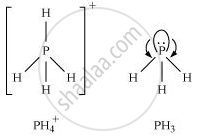Share

# Bond angle in PH4+ is higher than that in PH3. Why? - CBSE (Science) Class 12 - Chemistry

ConceptP - Block Group 15 Elements Oxides of Nitrogen

#### Question

Bond angle in PH_(4)^(+) is higher than that in PH3. Why?

#### Solution 1

In PH3, P is sp3 hybridized. Three orbitals are involved in bonding with three hydrogen atoms and the fourth one contains a lone pair. As lone pair-bond pair repulsion is stronger than bond pair-bond pair repulsion, the tetrahedral shape associated with sp3 bonding is changed to pyramidal. PH3 combines with a proton to form PH_4^(+) in which the lone pair is absent. Due to the absence of lone pair in PH_4^(+), there is no lone pair-bond pair repulsion. Hence, the bond angle in is higher than the bond angle in PH3#### Solution 2

P in PH3 is sp3-hybridized with 3 bond pairs and one lone pair around P. Due to stronger lp-bp repulsions than bp-bp repulsions, tetrahedral angle decreases from 109°28′ to 93.6°. As a result, PH3 is pyramidal. In PH4+, there are 4 bp’s and no lone pair. As a result, there are only identical bp-bp repulsions so that PH4+ assumes tetrahedral geometry and the bond angle is 109°28′.Hence, bond angle of PH4+ > bond angle of PH3

Is there an error in this question or solution?

#### APPEARS IN

NCERT Solution for Chemistry Textbook for Class 12 (2018 to Current)
Chapter 7: The p-block Elements
Q: 7 | Page no. 177

#### Video TutorialsVIEW ALL 

Solution Bond angle in PH4+ is higher than that in PH3. Why? Concept: P - Block Group 15 Elements - Oxides of Nitrogen.
S